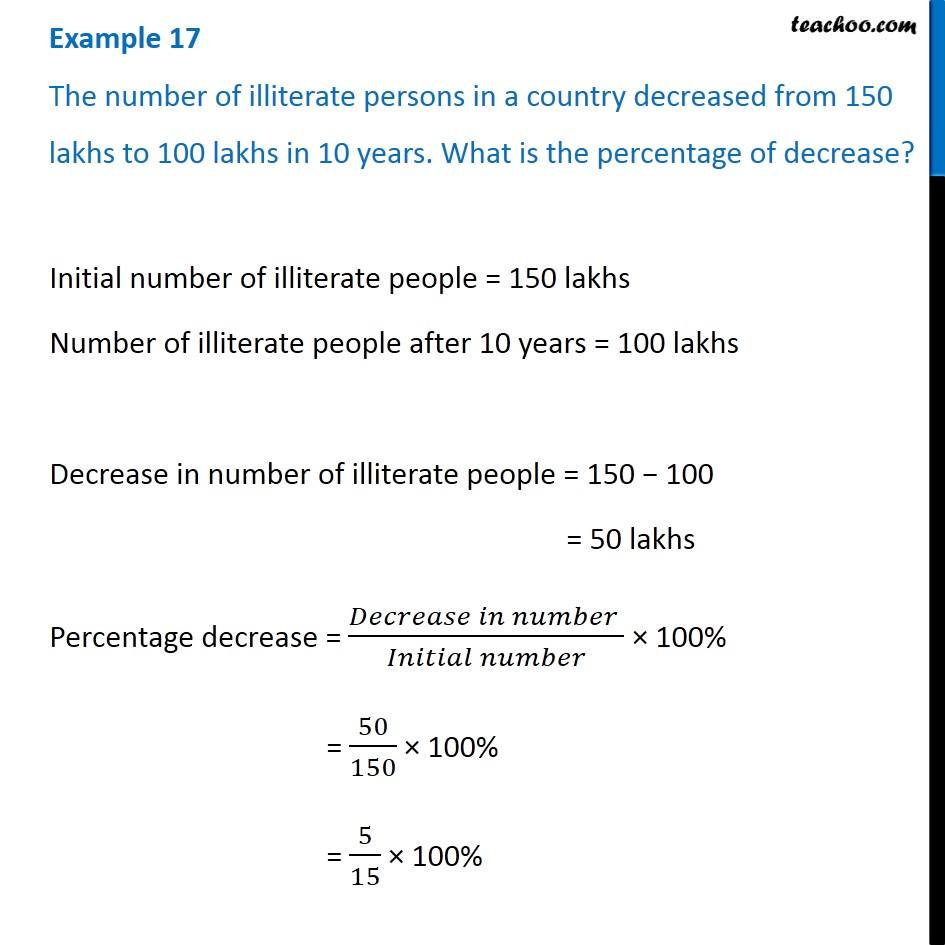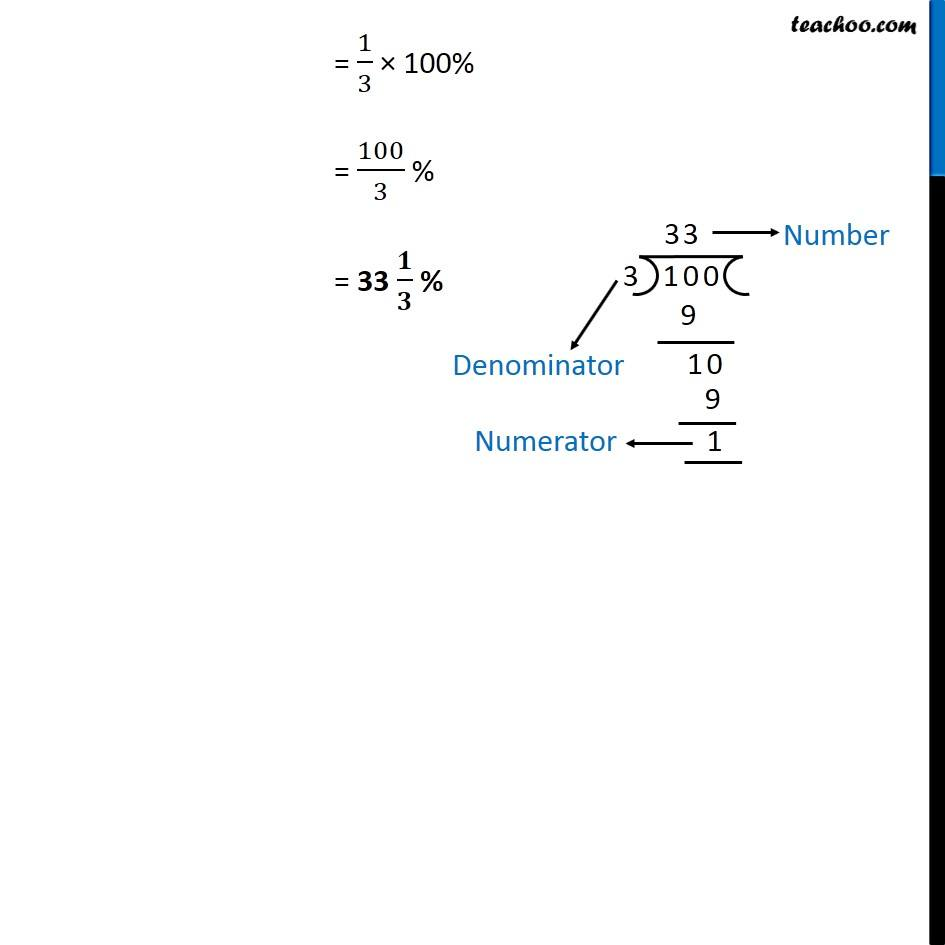Examples

Chapter 8 Class 7 Comparing Quantities
Serial order wise### Transcript

Example 17 The number of illiterate persons in a country decreased from 150 lakhs to 100 lakhs in 10 years. What is the percentage of decrease? Initial number of illiterate people = 150 lakhs Number of illiterate people after 10 years = 100 lakhs Decrease in number of illiterate people = 150 − 100 = 50 lakhs Percentage decrease = (𝐷𝑒𝑐𝑟𝑒𝑎𝑠𝑒 𝑖𝑛 𝑛𝑢𝑚𝑏𝑒𝑟 )/(𝐼𝑛𝑖𝑡𝑖𝑎𝑙 𝑛𝑢𝑚𝑏𝑒𝑟) × 100% = 50/150 × 100% = 5/15 × 100% = 1/3 × 100% = 100/3 % = 33 𝟏/𝟑 %